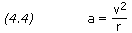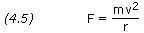﻿ Uniform Circular Motion | Aerospace Engineering

## Uniform Circular Motion

### Orbit Meccanics:

In the simple case of free fall, a particle accelerates toward the center of the Earth while moving in a straight line. The velocity of the particle changes in magnitude, but not in direction. In the case of uniform circular motion a particle moves in a circle with constant speed. The velocity of the particle changes continuously in direction, but not in magnitude. From Newton’s laws we see that since the direction of the velocity is changing, there is an acceleration. This acceleration, called centripetal acceleration is directed inward toward the center of the circle and is given bywhere v is the speed of the particle and r is the radius of the circle. Every accelerating particle must have a force acting on it, defined by Newton’s second law (F = ma). Thus, a particle undergoing uniform circular motion is under the influence of a force, called centripetal force, whose magnitude is given byThe direction of F at any instant must be in the direction of a at the same instant, that is radially inward.

A satellite in orbit is acted on only by the forces of gravity. The inward acceleration which causes the satellite to move in a circular orbit is the gravitational acceleration caused by the body around which the satellite orbits. Hence, the satellite’s centripetal acceleration is g, that is g = v2/r. From Newton’s law of universal gravitation we know that g = GM /r2. Therefore, by setting these equations equal to one another we find that, for a circular orbit,```PROBLEM

Calculate the velocity of an artificial satellite orbiting the
Earth in a circular orbit at an altitude of 200 km above the
Earth's surface.

SOLUTION,

From Basics Constants,

Radius of Earth = 6,378.140 km
GM of Earth = 3.986005×1014 m3/s2

Given:  r = (6,378.14 + 200) × 1,000 = 6,578,140 m

Equation (4.6),

v = SQRT[ GM / r ]
v = SQRT[ 3.986005×1014 / 6,578,140 ]
v = 7,784 m/s```